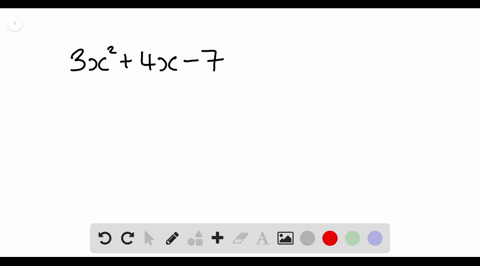Enroll in one of our FREE online STEM summer camps. Space is limited so join now!View Summer Courses### Factor each polynomial. If a polynomial cannot be…

02:46Other Schools

Need more help? Fill out this quick form to get professional live tutoring.

Get live tutoring
Problem 11

Factor each polynomial. If a polynomial cannot be factored, write prime. Factor out the greatest common factor as necessary.
$$y^{2}-4 y z-21 z^{2}$$

$y^{2}-4 y z-21 z^{2}=(y+3 z)(y-7 z)$

## Discussion

You must be signed in to discuss.

## Video Transcript

were asked a factor. This train a meal here. Why score tells us that both factors mustard with why and the negative 20 ones that tells us that it will be a number, Time said. And another number, Time said. And these two numbers must multiply together to be negative 21 and add together to be negative for. So if we list are factors of negative 21 we will get negative one comma, 21 negative, three comma seven Negative seven comma three and they give 21 comma one and out of these pears on Lee the negative seven comma three. Well, multiply. Sorry, we'll add together to be negative for So this tells us that are factors are going to be why minus seven said. And why? Plus three said.